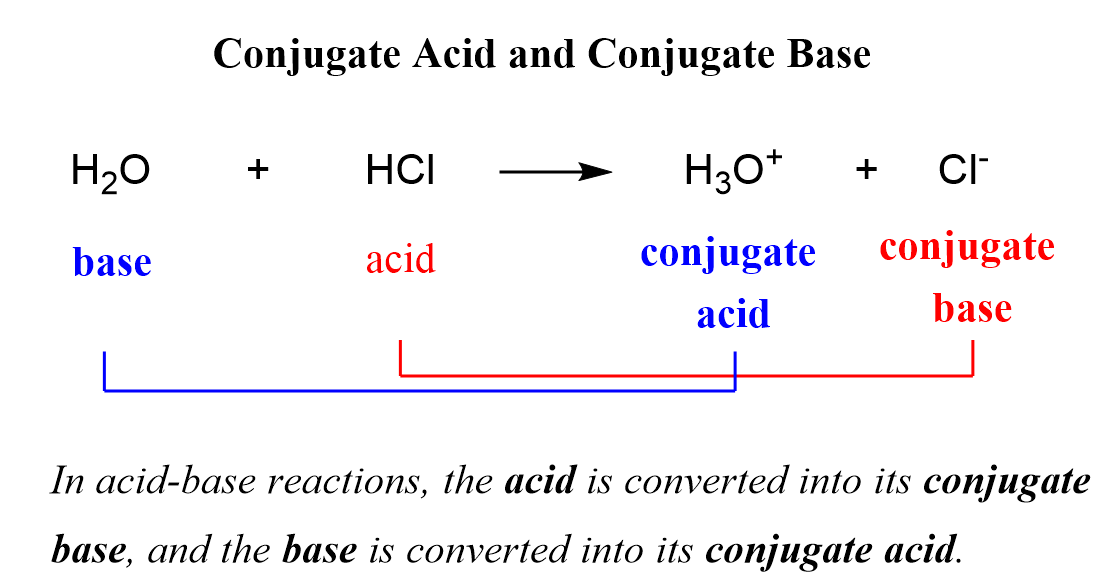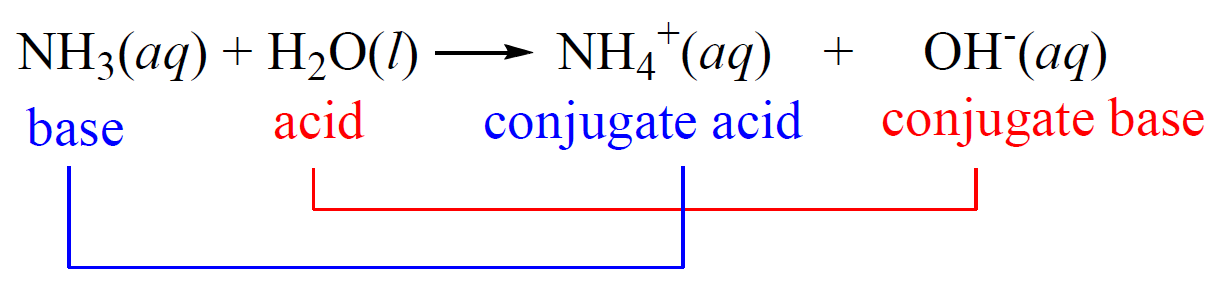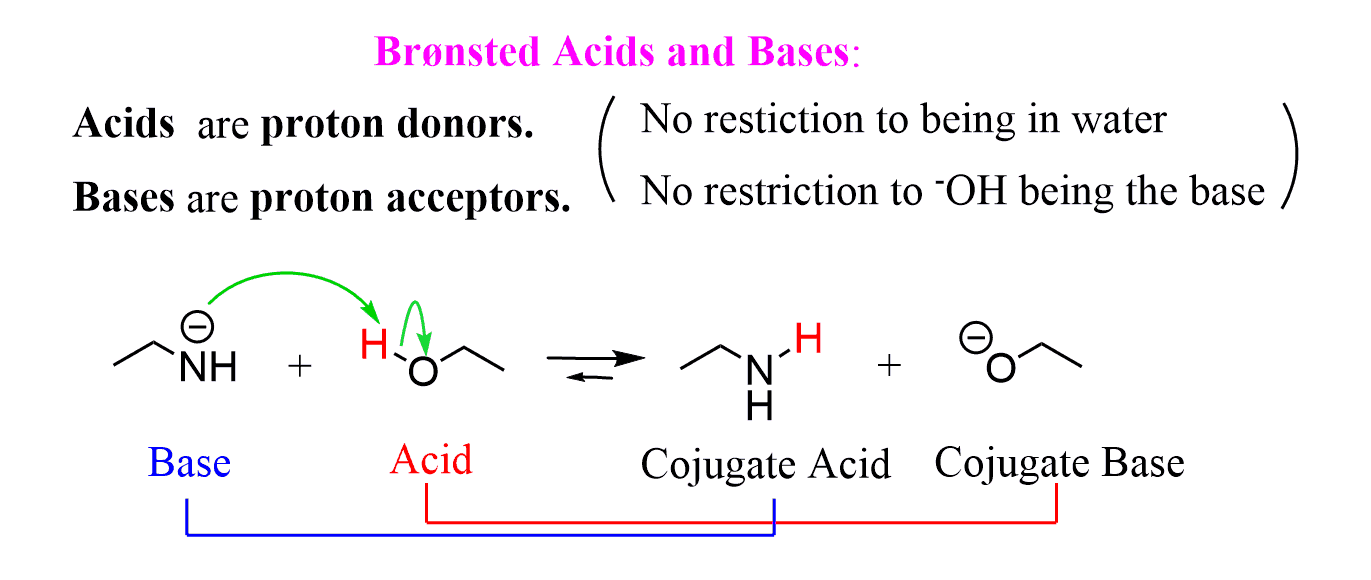## General Chemistry

In the previous post, we discussed the Arrhenius, Brønsted–Lowry, and Lewis definitions of acids and bases. One more concept in the Brønsted definition of acids and bases is the conjugate acid-base pair.

When the acid loses its proton, it becomes the conjugate base of the acid, and when the base accepts the proton, it becomes the conjugate acid of the base:So, why do we classify the products as acid or base?

One reason is that not all the reactions go to completion and therefore, an equilibrium is established where it is accepted to call the species on the left reactants and the ones on the right the products.

Remember, however, that the so-called products still react at equilibrium, and to avoid confusion in acid-base reactions, the terms conjugate acid, and conjugate base are used.

Acid-base reactions reach an equilibrium when a weak acid and base are used. For example, the reaction between ammonia and water is an example of a weak acid-base reaction and therefore, the ammonium ion (NH4+) is referred to as the conjugate acid, and the hydroxide ion as the conjugate base:Notice that we are not saying that the ammonium ion is always a conjugate acid and that the hydroxide ion is always a conjugate base. The classifications are given for the given reaction depending on the initial acid and the base.

The notation of conjugate acid and a base is also largely used in organic chemistry where it is often needed to predict the direction of the acid-base reaction:This is for later discussion if you are taking organic chemistry, however, you can read about it more here.

Check Also

1686019281